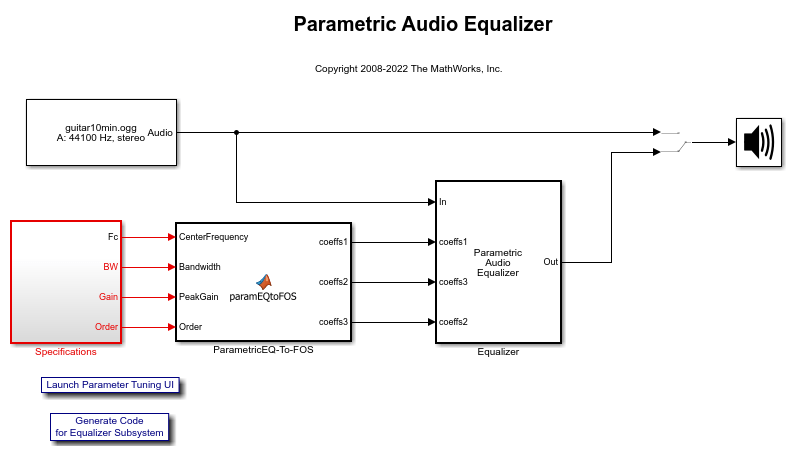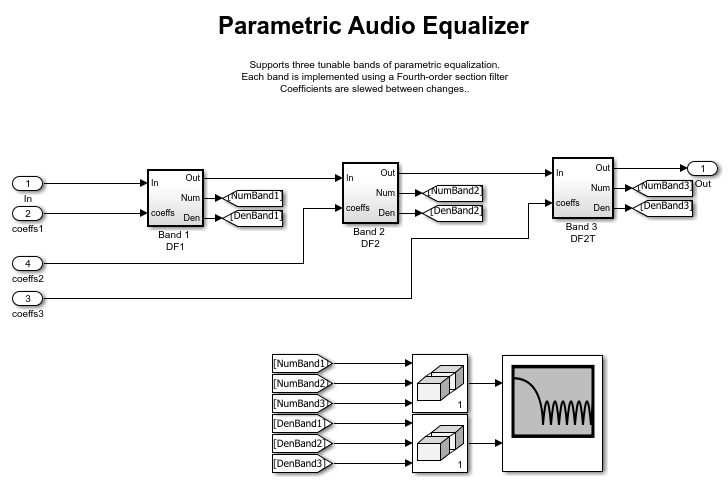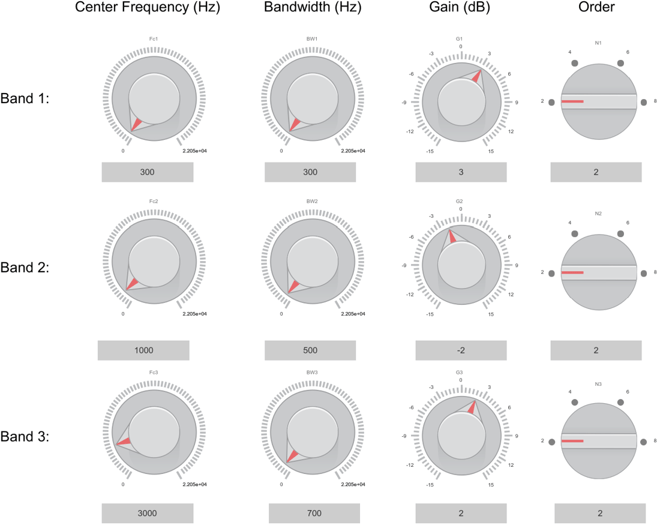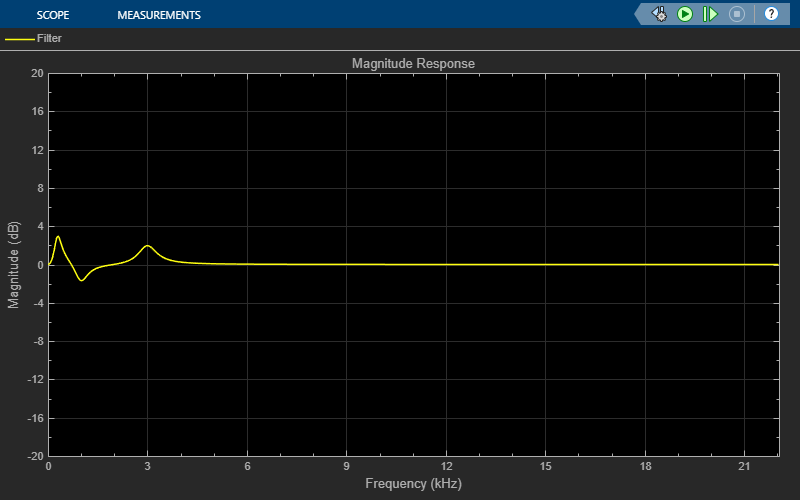# Parametric Audio Equalizer

This example shows how to model an algorithm specification for a three band parametric equalizer

### Introduction

Parametric equalizers are often used to adjust the frequency response of an audio system. For example, a parametric equalizer can be used to compensate for physical speakers which have peaks and dips at different frequencies.

The parametric equalizer algorithm in this example provides three fourth-order section filters whose coefficients can be adjusted to achieve a desired frequency response. A user interface (UI) is used in simulation to dynamically adjust filter coefficients and explore behavior.

This example describes how the parametric equalizer algorithm is specified and how the behavior can be explored through simulation.

Open and run the model.### Parametric Equalizer

In this example, the equalizer is implemented in the Equalizer subsystem of the model. In this subsystem, the input is passed through three cascaded bands of equalization. Coefficient changes within each band are smoothed through a leaky integrator before being passed into a Fourth-order Section Filter block. The Filter Visualizer block is used to visualize the magnitude response of the three cascaded bands.### Equalizer Specifications

This example allows tuning of each equalizer band's center frequency, bandwidth, peak (or dip) gain, and order. The bandwidth is defined at the arithmetic mean between the base of the filter (1 in this example) and the peak power value. The specifications of the three bands are in the Specifications subsystem. These specifications are converted to Fourth-order coefficients using a MATLAB Function block. The coefficients of a particular band are recomputed whenever any of that band's specifications are modified.

### User Interface

A UI designed to interact with the simulation is designed using Simulink dashboard blocks. This UI is provided with the model and can be launched by clicking the 'Launch Parameter Tuning UI' link. The UI allows you to tune the equalizer specifications and the results are reflected in the simulation instantly.### Exploring the Simulation

When you simulate the model, you can visualize the equalizer's response on a scope. The response is computed using a Dynamic Filter Visualizer which takes in the filter coefficients. The response changes as you tune the equalizer specifications. You can also listen to either the original or equalized audio by toggling the manual switch.### Generate C Code for the Equalizer Subsystem

To learn how to generate C code for the equalizer subsystem that is implemented using biquadratic sections based on the algorithm specifications, see the Code Generation for Parametric Audio Equalizer example.